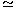# Electronics and Communication Engineering - Materials and Components - Discussion

4.

If k is Boltzmann's constant and T is absolute temp, then at room temp.

 [A]. kT0.025 eV [B]. kT0.5 eV [C]. kT1 eV [D]. kT100 eV

Explanation:

No answer description available for this question.

 Mohan Kumar said: (Oct 8, 2016) Thermal velocity is equal to the product of Boltzman Comtant *temperature/Q Thermal velocity = T/11600. At room temp., Thermal velocity = 273° + 27°/11600 = 300/11600 = 0.026 volt.

 Rohan Yaav said: (Aug 13, 2017) Thermal voltage=(K * T)/Q. K=boltzmann constant=1.38*10 to power -23. T= Room temperature=300 kelvin. Q= charge on one electron= 1.6 * 10 to power -19. Since the answers are given in eV we have to calculate using above formulae. Answer= ~0.025eV.